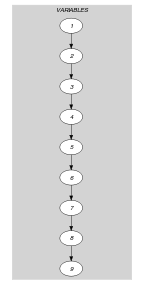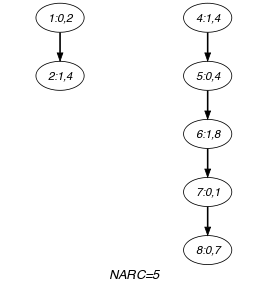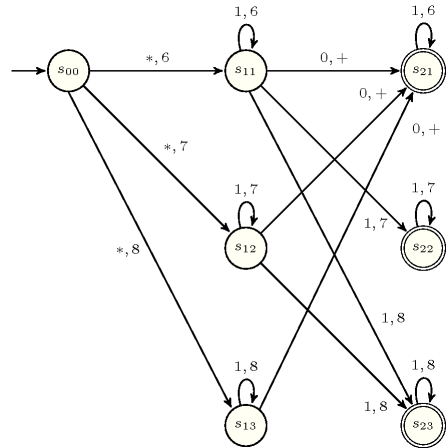## 5.188. increasing_nvalue_chain

Origin
Constraint

$\mathrm{𝚒𝚗𝚌𝚛𝚎𝚊𝚜𝚒𝚗𝚐}_\mathrm{𝚗𝚟𝚊𝚕𝚞𝚎}_\mathrm{𝚌𝚑𝚊𝚒𝚗}\left(\mathrm{𝙽𝚅𝙰𝙻},\mathrm{𝚅𝙰𝚁𝙸𝙰𝙱𝙻𝙴𝚂}\right)$

Arguments
 $\mathrm{𝙽𝚅𝙰𝙻}$ $\mathrm{𝚍𝚟𝚊𝚛}$ $\mathrm{𝚅𝙰𝚁𝙸𝙰𝙱𝙻𝙴𝚂}$ $\mathrm{𝚌𝚘𝚕𝚕𝚎𝚌𝚝𝚒𝚘𝚗}\left(𝚋-\mathrm{𝚍𝚟𝚊𝚛},\mathrm{𝚟𝚊𝚛}-\mathrm{𝚍𝚟𝚊𝚛}\right)$
Restrictions
 $\mathrm{𝙽𝚅𝙰𝙻}\ge \mathrm{𝚖𝚒𝚗}\left(1,|\mathrm{𝚅𝙰𝚁𝙸𝙰𝙱𝙻𝙴𝚂}|\right)$ $\mathrm{𝙽𝚅𝙰𝙻}\le |\mathrm{𝚅𝙰𝚁𝙸𝙰𝙱𝙻𝙴𝚂}|$ $\mathrm{𝚛𝚎𝚚𝚞𝚒𝚛𝚎𝚍}$$\left(\mathrm{𝚅𝙰𝚁𝙸𝙰𝙱𝙻𝙴𝚂},\left[𝚋,\mathrm{𝚟𝚊𝚛}\right]\right)$ $\mathrm{𝚅𝙰𝚁𝙸𝙰𝙱𝙻𝙴𝚂}.𝚋\ge 0$ $\mathrm{𝚅𝙰𝚁𝙸𝙰𝙱𝙻𝙴𝚂}.𝚋\le 1$
Purpose

For each consecutive pair of items $\mathrm{𝚅𝙰𝚁𝙸𝙰𝙱𝙻𝙴𝚂}\left[i\right],\mathrm{𝚅𝙰𝚁𝙸𝙰𝙱𝙻𝙴𝚂}\left[i+1\right]$ $\left(1\le i<|\mathrm{𝚅𝙰𝚁𝙸𝙰𝙱𝙻𝙴𝚂}|\right)$ of the $\mathrm{𝚅𝙰𝚁𝙸𝙰𝙱𝙻𝙴𝚂}$ collection at least one of the following conditions hold:

1. $\mathrm{𝚅𝙰𝚁𝙸𝙰𝙱𝙻𝙴𝚂}\left[i+1\right].𝚋=0$,

2. $\mathrm{𝚅𝙰𝚁𝙸𝙰𝙱𝙻𝙴𝚂}\left[i\right].\mathrm{𝚟𝚊𝚛}\le \mathrm{𝚅𝙰𝚁𝙸𝙰𝙱𝙻𝙴𝚂}\left[i+1\right].\mathrm{𝚟𝚊𝚛}$.

In addition, $\mathrm{𝙽𝚅𝙰𝙻}$ is equal to number of pairs of variables $\mathrm{𝚅𝙰𝚁𝙸𝙰𝙱𝙻𝙴𝚂}\left[i\right],\mathrm{𝚅𝙰𝚁𝙸𝙰𝙱𝙻𝙴𝚂}\left[i+1\right]$ $\left(1\le i<|\mathrm{𝚅𝙰𝚁𝙸𝙰𝙱𝙻𝙴𝚂}|\right)$ plus one, which verify at least one of the following conditions:

1. $\mathrm{𝚅𝙰𝚁𝙸𝙰𝙱𝙻𝙴𝚂}\left[i+1\right].𝚋=0$,

2. $\mathrm{𝚅𝙰𝚁𝙸𝙰𝙱𝙻𝙴𝚂}\left[i\right].\mathrm{𝚟𝚊𝚛}<\mathrm{𝚅𝙰𝚁𝙸𝙰𝙱𝙻𝙴𝚂}\left[i+1\right].\mathrm{𝚟𝚊𝚛}$.

Note that $\mathrm{𝚅𝙰𝚁𝙸𝙰𝙱𝙻𝙴𝚂}\left[1\right].𝚋$ is not referenced at all in the previous definition (i.e., its value does not influence at all the values assigned to the other variables).

Example
$\left(\begin{array}{c}6,〈\begin{array}{cc}𝚋-0\hfill & \mathrm{𝚟𝚊𝚛}-2,\hfill \\ 𝚋-1\hfill & \mathrm{𝚟𝚊𝚛}-4,\hfill \\ 𝚋-1\hfill & \mathrm{𝚟𝚊𝚛}-4,\hfill \\ 𝚋-1\hfill & \mathrm{𝚟𝚊𝚛}-4,\hfill \\ 𝚋-0\hfill & \mathrm{𝚟𝚊𝚛}-4,\hfill \\ 𝚋-1\hfill & \mathrm{𝚟𝚊𝚛}-8,\hfill \\ 𝚋-0\hfill & \mathrm{𝚟𝚊𝚛}-1,\hfill \\ 𝚋-0\hfill & \mathrm{𝚟𝚊𝚛}-7,\hfill \\ 𝚋-1\hfill & \mathrm{𝚟𝚊𝚛}-7\hfill \end{array}〉\hfill \end{array}\right)$

The $\mathrm{𝚒𝚗𝚌𝚛𝚎𝚊𝚜𝚒𝚗𝚐}_\mathrm{𝚗𝚟𝚊𝚕𝚞𝚎}_\mathrm{𝚌𝚑𝚊𝚒𝚗}$ constraint holds since:

1. The condition $\mathrm{𝚅𝙰𝚁𝙸𝙰𝙱𝙻𝙴𝚂}\left[i+1\right].𝚋=0\vee \mathrm{𝚅𝙰𝚁𝙸𝙰𝙱𝙻𝙴𝚂}\left[i\right].\mathrm{𝚟𝚊𝚛}\le \mathrm{𝚅𝙰𝚁𝙸𝙰𝙱𝙻𝙴𝚂}\left[i+1\right].\mathrm{𝚟𝚊𝚛}$ holds for every pair of adjacent items of the $\mathrm{𝚅𝙰𝚁𝙸𝙰𝙱𝙻𝙴𝚂}$ collection:

• For the pair $\left(\mathrm{𝚅𝙰𝚁𝙸𝙰𝙱𝙻𝙴𝚂}\left[1\right].\mathrm{𝚟𝚊𝚛},\mathrm{𝚅𝙰𝚁𝙸𝙰𝙱𝙻𝙴𝚂}\left[2\right].\mathrm{𝚟𝚊𝚛}\right)$ we have $\mathrm{𝚅𝙰𝚁𝙸𝙰𝙱𝙻𝙴𝚂}\left[1\right].\mathrm{𝚟𝚊𝚛}\le \mathrm{𝚅𝙰𝚁𝙸𝙰𝙱𝙻𝙴𝚂}\left[2\right].\mathrm{𝚟𝚊𝚛}$ $\left(2\le 4\right)$.

• For the pair $\left(\mathrm{𝚅𝙰𝚁𝙸𝙰𝙱𝙻𝙴𝚂}\left[2\right].\mathrm{𝚟𝚊𝚛},\mathrm{𝚅𝙰𝚁𝙸𝙰𝙱𝙻𝙴𝚂}\left[3\right].\mathrm{𝚟𝚊𝚛}\right)$ we have $\mathrm{𝚅𝙰𝚁𝙸𝙰𝙱𝙻𝙴𝚂}\left[2\right].\mathrm{𝚟𝚊𝚛}\le \mathrm{𝚅𝙰𝚁𝙸𝙰𝙱𝙻𝙴𝚂}\left[3\right].\mathrm{𝚟𝚊𝚛}$ $\left(4\le 4\right)$.

• For the pair $\left(\mathrm{𝚅𝙰𝚁𝙸𝙰𝙱𝙻𝙴𝚂}\left[3\right].\mathrm{𝚟𝚊𝚛},\mathrm{𝚅𝙰𝚁𝙸𝙰𝙱𝙻𝙴𝚂}\left[4\right].\mathrm{𝚟𝚊𝚛}\right)$ we have $\mathrm{𝚅𝙰𝚁𝙸𝙰𝙱𝙻𝙴𝚂}\left[3\right].\mathrm{𝚟𝚊𝚛}\le \mathrm{𝚅𝙰𝚁𝙸𝙰𝙱𝙻𝙴𝚂}\left[4\right].\mathrm{𝚟𝚊𝚛}$ $\left(4\le 4\right)$.

• For the pair $\left(\mathrm{𝚅𝙰𝚁𝙸𝙰𝙱𝙻𝙴𝚂}\left[4\right].\mathrm{𝚟𝚊𝚛},\mathrm{𝚅𝙰𝚁𝙸𝙰𝙱𝙻𝙴𝚂}\left[5\right].\mathrm{𝚟𝚊𝚛}\right)$ we have $\mathrm{𝚅𝙰𝚁𝙸𝙰𝙱𝙻𝙴𝚂}\left[5\right].𝚋=0$.

• For the pair $\left(\mathrm{𝚅𝙰𝚁𝙸𝙰𝙱𝙻𝙴𝚂}\left[5\right].\mathrm{𝚟𝚊𝚛},\mathrm{𝚅𝙰𝚁𝙸𝙰𝙱𝙻𝙴𝚂}\left[6\right].\mathrm{𝚟𝚊𝚛}\right)$ we have $\mathrm{𝚅𝙰𝚁𝙸𝙰𝙱𝙻𝙴𝚂}\left[5\right].\mathrm{𝚟𝚊𝚛}\le \mathrm{𝚅𝙰𝚁𝙸𝙰𝙱𝙻𝙴𝚂}\left[6\right].\mathrm{𝚟𝚊𝚛}$ $\left(4\le 8\right)$.

• For the pair $\left(\mathrm{𝚅𝙰𝚁𝙸𝙰𝙱𝙻𝙴𝚂}\left[6\right].\mathrm{𝚟𝚊𝚛},\mathrm{𝚅𝙰𝚁𝙸𝙰𝙱𝙻𝙴𝚂}\left[7\right].\mathrm{𝚟𝚊𝚛}\right)$ we have $\mathrm{𝚅𝙰𝚁𝙸𝙰𝙱𝙻𝙴𝚂}\left[7\right].𝚋=0$.

• For the pair $\left(\mathrm{𝚅𝙰𝚁𝙸𝙰𝙱𝙻𝙴𝚂}\left[7\right].\mathrm{𝚟𝚊𝚛},\mathrm{𝚅𝙰𝚁𝙸𝙰𝙱𝙻𝙴𝚂}\left[8\right].\mathrm{𝚟𝚊𝚛}\right)$ we have $\mathrm{𝚅𝙰𝚁𝙸𝙰𝙱𝙻𝙴𝚂}\left[8\right].𝚋=0$.

• For the pair $\left(\mathrm{𝚅𝙰𝚁𝙸𝙰𝙱𝙻𝙴𝚂}\left[8\right].\mathrm{𝚟𝚊𝚛},\mathrm{𝚅𝙰𝚁𝙸𝙰𝙱𝙻𝙴𝚂}\left[9\right].\mathrm{𝚟𝚊𝚛}\right)$ we have $\mathrm{𝚅𝙰𝚁𝙸𝙰𝙱𝙻𝙴𝚂}\left[8\right].\mathrm{𝚟𝚊𝚛}\le \mathrm{𝚅𝙰𝚁𝙸𝙰𝙱𝙻𝙴𝚂}\left[9\right].\mathrm{𝚟𝚊𝚛}$ $\left(7\le 7\right)$.

2. $\mathrm{𝙽𝚅𝙰𝙻}$ is equal to number of pairs of variables $\mathrm{𝚅𝙰𝚁𝙸𝙰𝙱𝙻𝙴𝚂}\left[i\right],\mathrm{𝚅𝙰𝚁𝙸𝙰𝙱𝙻𝙴𝚂}\left[i+1\right]$ $\left(1\le i<|\mathrm{𝚅𝙰𝚁𝙸𝙰𝙱𝙻𝙴𝚂}|\right)$ plus one which verify at least $\mathrm{𝚅𝙰𝚁𝙸𝙰𝙱𝙻𝙴𝚂}\left[i+1\right].𝚋=0\vee \mathrm{𝚅𝙰𝚁𝙸𝙰𝙱𝙻𝙴𝚂}\left[i\right].\mathrm{𝚟𝚊𝚛}<\mathrm{𝚅𝙰𝚁𝙸𝙰𝙱𝙻𝙴𝚂}\left[i+1\right].\mathrm{𝚟𝚊𝚛}$. Beside the plus one, the following five pairs contribute for 1 in $\mathrm{𝙽𝚅𝙰𝙻}$:

• For the pair $\left(\mathrm{𝚅𝙰𝚁𝙸𝙰𝙱𝙻𝙴𝚂}\left[1\right].\mathrm{𝚟𝚊𝚛},\mathrm{𝚅𝙰𝚁𝙸𝙰𝙱𝙻𝙴𝚂}\left[2\right].\mathrm{𝚟𝚊𝚛}\right)$ we have $\mathrm{𝚅𝙰𝚁𝙸𝙰𝙱𝙻𝙴𝚂}\left[1\right].\mathrm{𝚟𝚊𝚛}\le \mathrm{𝚅𝙰𝚁𝙸𝙰𝙱𝙻𝙴𝚂}\left[2\right].\mathrm{𝚟𝚊𝚛}$ $\left(2<4\right)$.

• For the pair $\left(\mathrm{𝚅𝙰𝚁𝙸𝙰𝙱𝙻𝙴𝚂}\left[4\right].\mathrm{𝚟𝚊𝚛},\mathrm{𝚅𝙰𝚁𝙸𝙰𝙱𝙻𝙴𝚂}\left[5\right].\mathrm{𝚟𝚊𝚛}\right)$ we have $\mathrm{𝚅𝙰𝚁𝙸𝙰𝙱𝙻𝙴𝚂}\left[5\right].𝚋=0$.

• For the pair $\left(\mathrm{𝚅𝙰𝚁𝙸𝙰𝙱𝙻𝙴𝚂}\left[5\right].\mathrm{𝚟𝚊𝚛},\mathrm{𝚅𝙰𝚁𝙸𝙰𝙱𝙻𝙴𝚂}\left[6\right].\mathrm{𝚟𝚊𝚛}\right)$ we have $\mathrm{𝚅𝙰𝚁𝙸𝙰𝙱𝙻𝙴𝚂}\left[5\right].\mathrm{𝚟𝚊𝚛}\le \mathrm{𝚅𝙰𝚁𝙸𝙰𝙱𝙻𝙴𝚂}\left[6\right].\mathrm{𝚟𝚊𝚛}$ $\left(4<8\right)$.

• For the pair $\left(\mathrm{𝚅𝙰𝚁𝙸𝙰𝙱𝙻𝙴𝚂}\left[6\right].\mathrm{𝚟𝚊𝚛},\mathrm{𝚅𝙰𝚁𝙸𝙰𝙱𝙻𝙴𝚂}\left[7\right].\mathrm{𝚟𝚊𝚛}\right)$ we have $\mathrm{𝚅𝙰𝚁𝙸𝙰𝙱𝙻𝙴𝚂}\left[7\right].𝚋=0$.

• For the pair $\left(\mathrm{𝚅𝙰𝚁𝙸𝙰𝙱𝙻𝙴𝚂}\left[7\right].\mathrm{𝚟𝚊𝚛},\mathrm{𝚅𝙰𝚁𝙸𝙰𝙱𝙻𝙴𝚂}\left[8\right].\mathrm{𝚟𝚊𝚛}\right)$ we have $\mathrm{𝚅𝙰𝚁𝙸𝙰𝙱𝙻𝙴𝚂}\left[8\right].𝚋=0$.

Typical
 $|\mathrm{𝚅𝙰𝚁𝙸𝙰𝙱𝙻𝙴𝚂}|>1$ $\mathrm{𝚛𝚊𝚗𝚐𝚎}$$\left(\mathrm{𝚅𝙰𝚁𝙸𝙰𝙱𝙻𝙴𝚂}.𝚋\right)>1$ $\mathrm{𝚛𝚊𝚗𝚐𝚎}$$\left(\mathrm{𝚅𝙰𝚁𝙸𝙰𝙱𝙻𝙴𝚂}.\mathrm{𝚟𝚊𝚛}\right)>1$
Keywords
Arc input(s)

$\mathrm{𝚅𝙰𝚁𝙸𝙰𝙱𝙻𝙴𝚂}$

Arc generator
$\mathrm{𝑃𝐴𝑇𝐻}$$↦\mathrm{𝚌𝚘𝚕𝚕𝚎𝚌𝚝𝚒𝚘𝚗}\left(\mathrm{𝚟𝚊𝚛𝚒𝚊𝚋𝚕𝚎𝚜}\mathtt{1},\mathrm{𝚟𝚊𝚛𝚒𝚊𝚋𝚕𝚎𝚜}\mathtt{2}\right)$

Arc arity
Arc constraint(s)
$\mathrm{𝚟𝚊𝚛𝚒𝚊𝚋𝚕𝚎𝚜}\mathtt{2}.𝚋=0\vee \mathrm{𝚟𝚊𝚛𝚒𝚊𝚋𝚕𝚎𝚜}\mathtt{1}.\mathrm{𝚟𝚊𝚛}\le \mathrm{𝚟𝚊𝚛𝚒𝚊𝚋𝚕𝚎𝚜}\mathtt{2}.\mathrm{𝚟𝚊𝚛}$
Graph property(ies)
$\mathrm{𝐍𝐀𝐑𝐂}$$=|\mathrm{𝚅𝙰𝚁𝙸𝙰𝙱𝙻𝙴𝚂}|-1$

Arc input(s)

$\mathrm{𝚅𝙰𝚁𝙸𝙰𝙱𝙻𝙴𝚂}$

Arc generator
$\mathrm{𝑃𝐴𝑇𝐻}$$↦\mathrm{𝚌𝚘𝚕𝚕𝚎𝚌𝚝𝚒𝚘𝚗}\left(\mathrm{𝚟𝚊𝚛𝚒𝚊𝚋𝚕𝚎𝚜}\mathtt{1},\mathrm{𝚟𝚊𝚛𝚒𝚊𝚋𝚕𝚎𝚜}\mathtt{2}\right)$

Arc arity
Arc constraint(s)
$\mathrm{𝚟𝚊𝚛𝚒𝚊𝚋𝚕𝚎𝚜}\mathtt{2}.𝚋=0\vee \mathrm{𝚟𝚊𝚛𝚒𝚊𝚋𝚕𝚎𝚜}\mathtt{1}.\mathrm{𝚟𝚊𝚛}<\mathrm{𝚟𝚊𝚛𝚒𝚊𝚋𝚕𝚎𝚜}\mathtt{2}.\mathrm{𝚟𝚊𝚛}$
Graph property(ies)
$\mathrm{𝐍𝐀𝐑𝐂}$$=\mathrm{𝙽𝚅𝙰𝙻}-1$

Graph model

Parts (A) and (B) of Figure 5.188.1 respectively show the initial and final graph associated with the second graph constraint of the Example slot. Since we use the $\mathrm{𝐍𝐀𝐑𝐂}$ graph property the arcs of the final graph are stressed in bold.

##### Figure 5.188.1. Initial and final graph of the $\mathrm{𝚒𝚗𝚌𝚛𝚎𝚊𝚜𝚒𝚗𝚐}_\mathrm{𝚗𝚟𝚊𝚕𝚞𝚎}_\mathrm{𝚌𝚑𝚊𝚒𝚗}$ constraint(a) (b)
Automaton

Without loss of generality, assume that the collection $\mathrm{𝚅𝙰𝚁𝙸𝙰𝙱𝙻𝙴𝚂}$ contains at least one variable (i.e., $|\mathrm{𝚅𝙰𝚁𝙸𝙰𝙱𝙻𝙴𝚂}|\ge 1$). Let $l$, $m$, $n$, $\mathrm{𝑚𝑖𝑛}$ and $\mathrm{𝑚𝑎𝑥}$ respectively denote the minimum and maximum possible value of variable $\mathrm{𝙽𝚅𝙰𝙻}$, the number of items of the collection $\mathrm{𝚅𝙰𝚁𝙸𝙰𝙱𝙻𝙴𝚂}$, the smallest value that can be assigned to $\mathrm{𝚅𝙰𝚁𝙸𝙰𝙱𝙻𝙴𝚂}\left[i\right].\mathrm{𝚟𝚊𝚛}$ $\left(1\le i\le n\right)$, and the largest value that can be assigned to $\mathrm{𝚅𝙰𝚁𝙸𝙰𝙱𝙻𝙴𝚂}\left[i\right].\mathrm{𝚟𝚊𝚛}$ $\left(1\le i\le n\right)$. Let $s=\mathrm{𝑚𝑎𝑥}-\mathrm{𝑚𝑖𝑛}+1$ denote the total number of potential values. Clearly, the maximum value of $\mathrm{𝙽𝚅𝙰𝙻}$ cannot exceed the quantity $d=min\left(m,n\right)$. The states of the automaton that only accepts solutions of the $\mathrm{𝚒𝚗𝚌𝚛𝚎𝚊𝚜𝚒𝚗𝚐}_\mathrm{𝚗𝚟𝚊𝚕𝚞𝚎}_\mathrm{𝚌𝚑𝚊𝚒𝚗}$ constraint can be defined in the following way:

• We have an initial state labelled by ${s}_{00}$.

• We have $d·s$ states labelled by ${s}_{ij}$ $\left(1\le i\le d,1\le j\le s\right)$.

Terminal states depend on the possible values of variable $\mathrm{𝙽𝚅𝙰𝙻}$ and correspond to those states ${s}_{ij}$ such that $i$ is a possible value for variable $\mathrm{𝙽𝚅𝙰𝙻}$. Note that we assume no further restriction on the domain of $\mathrm{𝙽𝚅𝙰𝙻}$ (otherwise the set of accepting states needs to be reduced in order to reflect the current set of possible values of $\mathrm{𝙽𝚅𝙰𝙻}$).

Transitions of the automaton are labelled by a pair of values $\left(\alpha ,\beta \right)$ and correspond to a condition of the form $\mathrm{𝚅𝙰𝚁𝙸𝙰𝙱𝙻𝙴𝚂}\left[i\right].𝚋=\alpha \wedge \mathrm{𝚅𝙰𝚁𝙸𝙰𝙱𝙻𝙴𝚂}\left[i\right].\mathrm{𝚟𝚊𝚛}=\beta$, $\left(1\le i\le n\right)$. Characters $*$ and $+$ respectively represent all values in $\left\{0,1\right\}$ and all values in $\left\{\mathrm{𝑚𝑖𝑛},\mathrm{𝑚𝑖𝑛}+1,\cdots ,\mathrm{𝑚𝑎𝑥}\right\}$. Four classes of transitions are respectively defined in the following way:

1. There is a transition, labelled by the pair $\left(*,\mathrm{𝑚𝑖𝑛}+j-1\right)$, from the initial state ${s}_{00}$ to the state ${s}_{1j}$ $\left(1\le j\le s\right)$. We use the $*$ character since $\mathrm{𝚅𝙰𝚁𝙸𝙰𝙱𝙻𝙴𝚂}\left[1\right].𝚋$ is not use at all in the definition of the $\mathrm{𝚒𝚗𝚌𝚛𝚎𝚊𝚜𝚒𝚗𝚐}_\mathrm{𝚗𝚟𝚊𝚕𝚞𝚎}_\mathrm{𝚌𝚑𝚊𝚒𝚗}$ constraint.

2. There is a loop, labelled by the pair $\left(1,\mathrm{𝑚𝑖𝑛}+j-1\right)$ for every state ${s}_{ij}$ $\left(1\le i\le d,1\le j\le s\right)$.

3. $\forall i\in \left[1,d-1\right],\forall j\in \left[1,s\right],\forall k\in \left[j+1,s\right]$ there is a transition labelled by the pair $\left(1,\mathrm{𝑚𝑖𝑛}+k-1\right)$ from ${s}_{ij}$ to ${s}_{i+1k}$.

4. $\forall i\in \left[1,d-1\right],\forall j\in \left[1,s\right]$ there is a transition labelled by the pair $\left(0,+\right)$ from ${s}_{ij}$ to ${s}_{i+11}$.

##### Figure 5.188.2. Automaton of the $\mathrm{𝚒𝚗𝚌𝚛𝚎𝚊𝚜𝚒𝚗𝚐}_\mathrm{𝚗𝚟𝚊𝚕𝚞𝚎}_\mathrm{𝚌𝚑𝚊𝚒𝚗}$ constraint under the hypothesis that all variables are assigned a value in $\left\{6,7,8\right\}$ and that $\mathrm{𝙽𝚅𝙰𝙻}$ is equal to 2. The character $*$ on a transition corresponds to a 0 or to a 1 and the $+$ corresponds to a 6, 7 or 8.